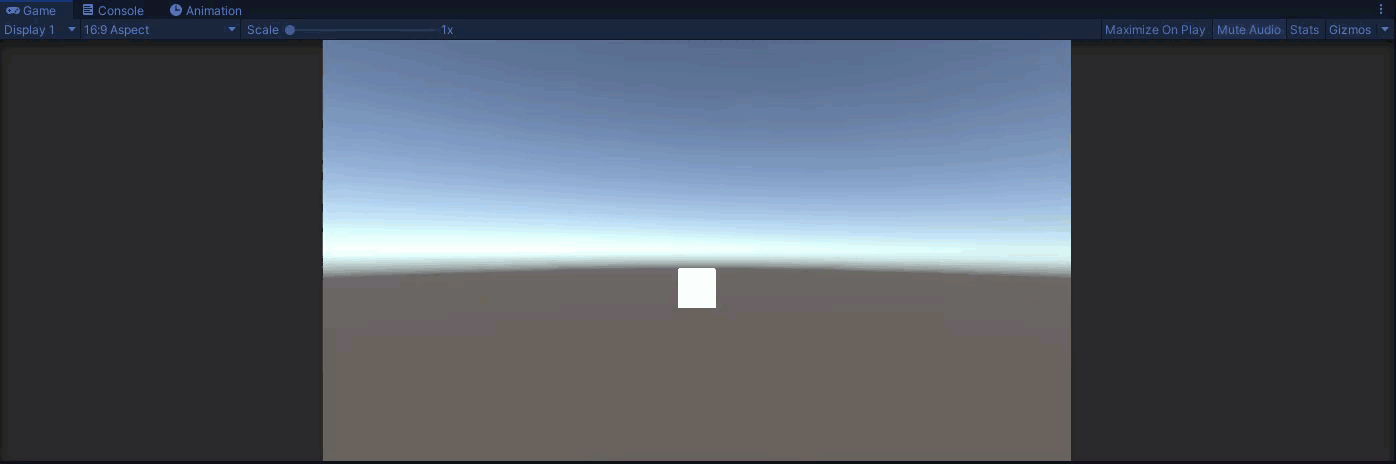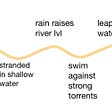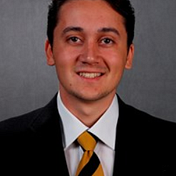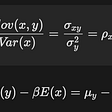# Statcast Data Manipulation in R

`install.packages("dplyr")library(dplyr)setwd("~/PATH TO FOLDER WITH CSV FILE")statcast_data <- read.csv("mlb_2020_statcast_pitcher.csv")`

# Filtering, Selecting, and Arranging Data

`NL_ROY <- statcast_data %>%   filter(player_name == "Devin Williams")NL_CY <- statcast_data %>%  filter(player_name %in% c("Trevor Bauer", "Jacob deGrom", "Yu Darvish"))`
`new_data_frame <- old_data_frame %>%  dplyr function`
`ROY_pitch_info <- NL_ROY %>%  select(player_name, pitch_type, release_speed, release_spin_rate)`
`ROY_columns <- NL_ROY %>%  select(player_name, pitch_type:release_speed)`
`ROY_release_speed <- NL_ROY %>%  select(player_name, pitch_type, release_speed, release_spin_rate) %>%  arrange(desc(release_speed))`
`ROY_FF <- NL_ROY %>%  select(player_name, pitch_type, release_speed, release_spin_rate) %>%  filter(pitch_type == "FF") %>%  arrange(desc(release_speed))`

# Grouping, Summarizing, and Mutating Data

`ROY_pitch_count <- NL_ROY %>%  group_by(pitch_type) %>%  summarize('pitch_count' = n())`
`summarize('COLUMN NAME' = FUNCTION())`
`ROY_avg_velos <- NL_ROY %>%  group_by(pitch_type) %>%  summarize('pitch_count' = n(),            'average_velocity' = mean(release_speed, na.rm = TRUE))ROY_range_velos <- NL_ROY %>%  group_by(pitch_type) %>%  summarize('pitch_count' = n(),            'min_velocity' = min(release_speed, na.rm = TRUE),            'max_velocity' = max(release_speed, na.rm = TRUE))`
`CY_FF_ranks <- NL_CY %>%  filter(pitch_type == "FF") %>%  group_by(player_name, pitch_type) %>%  summarize('average_velocity' = mean(release_speed, na.rm = TRUE),            'average_spin' = mean(release_spin_rate, na.rm = TRUE)) %>%  mutate('bauer_units' = round(average_spin/average_velocity,1))`

# Wrapping It Up

--

--

--

## More from Sam Bornstein

Driveline Baseball Operations Analyst

Love podcasts or audiobooks? Learn on the go with our new app.

## Introduction to the Topos Reliable Broadcast## The Empty Unity Scene## Design 6400 3/28 Journal## Implementing an R script in Google Cloud Run## ETHAmsterdam 2022: ETHGlobal is Back!## Playing with the Dark (language) and Gradle configuration caching## Sam Bornstein

Driveline Baseball Operations Analyst

## Simple Linear Regression with basic R## A Detailed Overview of Apply-Family Functions in R## What does ~. mean in R?